Considerations on the Appropriate Level of Protection,

Acceptable Level of Risk and Phytosanitary Measures

Ricardo Sgrillo

CEPEC/CEPLAC

Rod. Ilheus-Itabuna, km 22

45650-000 Ilheus, BA, Brazil

This paper represents the views of the author and not necessarily those of his country and/or organization.

Introduction

The concepts of Appropriate Level of Protection (ALP) and Acceptable Level of Risk (ALR) are important topics of discussion in national and international organizations concerned with the harmonization of sanitary and phytosanitary measures.The purpose of this paper is to contribute to these discussions with one explanation of these concepts as they may be interpreted for the establishment of phytosanitary measures as illustrated in Figure 1:

Figure 1.Process for the establishment of phytosanitary measures.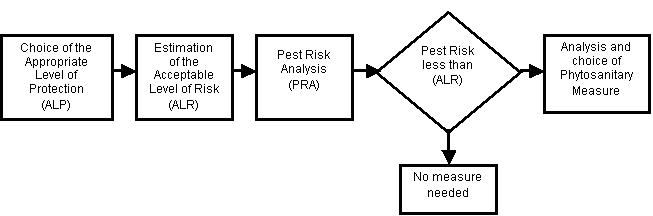1.Appropriate Level of Protection

The first box in Figure 1 represents the choice of the appropriate level of protection, a sovereign right of each country. For phytosanitary purposes, the appropriate level of protection is the level of protection that a country decides is necessary to protect plant health and life against the harmful effects of plant pests.This level of protection is achieved by reducing the risk of pest establishment to specified levels, the acceptable level of risk

To stipulate a level of protection for quarantine pests, it is first necessary to relate this to the pest risk, which in turn is related to the probability of pest establishment.This raises two key questions:

(1) How many pests are required to establish; and,

(2) What prevalence of pests will be allowed in a lot, consignment, year, or some other unit measurement?

The answer to the first question depends on many conditions, including biological and other factors, but the intuitive answer to the second question is zero.No country wants to accept shipments that are contaminated with pests.However, according to the principle of managed risk, zero risk is not possible.Even the prohibition of trade is not a zero-risk option (prohibition may actually increase risk because it can increase the motivation for smuggling).

Recognizing that zero risk is not an option and also that some level of pest prevalence is required for pest introduction, countries desire a level close to zero.And what is the meaning of "as close to zero as possible"?To discuss these points, it is important to first understand the important role of probability analysis.

Two key concepts need to be defined at the outset:

unit risk:the probability of introduction of a quarantine pest through the importation of one unit of the product during a specific period of time.

product risk:the probability of introduction of a quarantine pest through the importation of all units of one product, from one origin, during a specific period of time

The importation risk is estimated by multiplying the unit risk by the number of all the units of the product.The result represents the number of expected introductions per time unit.The reciprocal of the importation risk (1/risk) expresses the expected period of time between two introductions, which may be called the introduction period.Each case may be described by the unit introduction period and product introduction period.Using one year as the time unit, and assuming a risk of 0.01, we would expect one introduction each 100 years (1/0.01).Table 1 shows some of the relationships between unit risk, product risk and correspondent introduction periods where there are 100,000 total units in the consignments in one year.

Table 1. Relationship between unit risk, product risk and corresponding introduction periods.

 Unit risk Unit introduction period (Years) Product risk1 Product introduction period(Years) 1 1 100,000 0.00001 (one introduction each 5.2 minutes) 0.1 10 10,000 0.0001 (one introduction each 52 minutes) 0.01 100 1,000 0.001 (one introduction each 8.7 hours) 0.001 1000 100 0.01 (one introduction each 3,6 days) 0.0001 10,000 10 0.1 (one introduction each 36.5 days) 0.00001 100,000 1 1 (one introduction each year) 0.000001 1,000,000 0,1 10 (one introduction each 10 years) 0.0000001 10,000,000 0,01 100 0.00000001 100,000,000 0,001 1,000 0.000000001 1,000,000,000 0,0001 10,000 0.0000000001 10,000,000,000 0,00001 100,000 0.00000000001 100,000,000,000 0,000001 1,000,000 0.000000000001 1,000,000,000,000 0,0000001 10,000,000 0.0000000000001 10,000,000,000,000 0,00000001 100,000,000

1 Number of expected introductions in one year, considering the importation of 100,000 units

Each row in Table 1 represents a different level of product risk and a corresponding introduction period which also represents a level of protection.Some conclusions that may be drawn from the Table include:

§The level of protection is inversely proportional to the risk but it is not the risk itself.

§Zero risk (or full protection) is not practically achievable because there is always some likelihood of pest introduction if the quarantine pest is present in the origin.

§More protection is attained with lower risk.

§The risk of introduction increases with the increasing number of imports, implying that a country could perhaps require an annual quota for importation to maintain an acceptable level of risk (although technically justified, the practical implications of implementing such a requirement may be difficult).

So what is the meaning of "close to zero"?Is 0.00001 or the probability one introduction each 100,000 years close to zero?Or is it the probability of one introduction every 500 years?The main point to note at this stage is that although countries have the sovereign right to choose their appropriate level of protection, it is difficult to understand what this means unless it is expressed quantitatively in relation to an acceptable level of risk.This is consistent with the principle of transparency.

One way to express the level of protection numerically is as log10 of the product introduction period: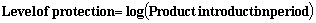Equation 1

Table 2 demonstrates the relationship of the product introduction period and product risk to the level of protection associated with representative levels from -1 to 6.As an example, a country may decide that 3 is an appropriate level of protection.This means that it accepts a product risk of 0.001, which is equivalent to one introduction every 1,000 years.This does not mean there will be an introduction every 1,000 years, but rather that this is the mean of a probability distribution with some probability that an introduction will occur in the first year and likewise a probability that introduction will occur in 999 years or later.

Table 2.Level of Protection in relation to product introduction period and product risk

 Level of Protection Product introduction period Product risk (introductions per time unit) -1 0.1 10 0 1 1 1 10 0.1 2 100 0.01 3 1,000 0.001 4 10,000 0.0001 5 100,000 0.00001 6 1,000,000 0.000001

The decision to specify an appropriate level of protection may not be based only on biological criteria but could also account for political, social, and economic aspects.For example:

·The country produces less than is needed and therefore has to import to supply the market (pushes ALP down)

·The country will lose international markets through the establishment of the pest (pushes ALP up)

·The importation would lower the price of the product, reducing the demand pressure and helping to reduce inflation pressure (pushes ALP down)

·The establishment of the pest will increase the production costs and the product would loose competitiveness (pushes ALP down)

·The national industry will have to improve the competitiveness of its product by improving the quality and decreasing the price (pushes ALP down)

·New employment would be generated through the need for handling a greater volume of the product (pushes ALP down).

· The crop in the importing country has strategic economic and social importance and the establishment of a new pest would have catastrophic consequences (strongly pushes ALP up)

2. Acceptable Level of Risk

The second box in Figure 1 refers to the estimation of the acceptable level of risk.This should be a straightforward procedure.From Equation 1 we can estimate the acceptable level of risk based on the appropriate level of protection and from the number of units to be imported: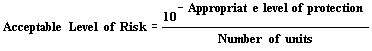Equation 2

For example, if a country establishes its appropriate level of protection as 2.7 (one introduction each 500 years), and intends to import 2,000 units, then the acceptable level of risk is 0.000001.This ALR refers to the unit risk.

3.Pest Risk Analysis

The next box in Figure 1 refers to pest risk analysis.This is a well-known procedure that does not require discussion in this paper.It is only necessary to note that a quantitative PRA would generate a probability of introduction and the statistics associated with it.If this probability is less then the acceptable level of risk, no measure should be required.

4.Phytosanitary Measure

The last box in Figure 1 represents the analysis and choice of phytosanitary measures.This is also a straightforward procedure but it requires that two additional concepts are defined:

risk of failure (of the measure):the proportion of the pest population that is not removed from the product by the phytosanitary measure.

efficacy (of the measure):the percentage of the population that is removed from the product by the phytosanitary measure.Sampling and experimentation are used to estimate efficacy.

The relationship between these variables is expressed in Equation 3: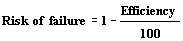Equation 3

The objective is to find a measure whose risk of failure multiplied by the probability of introduction (as estimated by PRA) will be equal or lower than the acceptable level of risk.This may be called the minimum required efficacy and the corresponding risk of failure would be the maximum allowable risk of failure.These concepts, together with Equation 3 are used to generate equation 4, where the minimum required efficacy may be estimated from the acceptable level of risk and from the probability of introduction: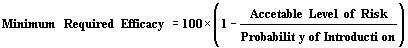Equation 4After this point it is necessary to compare the minimum required efficacy (or the maximum allowable risk of failure) with the actual efficacies (or risk of failure) of the available phytosanitary measures and to choose the most appropriate measure on this basis, considering also the need to choose the least trade restrictive measure if there are equivalent options.An economic analysis may also be useful for further evaluating measures where there are multiple options.

5. Example

To demonstrate the application of the above concepts in practice, assume a hypothetical situation where:

§Country A wants to export 20,000 boxes/year of a product to country B.

§Country B is concerned with a quarantine pest that occurs in country A and is known to be associated with the product to be exported.

The task is to choose appropriate measures based on a specified level of protection and calculations made from data supplied through PRA.

5.1. Appropriate Level of Protection (ALP) for country B:

Taking into account relevant economic, social and political factors, country B stipulates an appropriated level of protection of 2.48.The expected period of time between two introductions is 300 years and this is considered satisfactory for country B.

5.2. Acceptable Level of Risk (ALR)

The acceptable level of risk, estimated with Equation 2 is: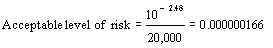5.3. Pest Risk Analysis

Country B developed a quantitative PRA with the results found in Table 3.

Table 3. Results of the quantitative PRA

 Probability of infestation at the origin Probability of failure of inspection in country A Probability of survival during handling and transport Probability of failure of inspection in country B Probability the pest is movedto a suitable environment for establishment Final Probability of introduction for each imported box (0.9) (0.1) (0.8) (0.1) (0.2) = 0.00144

Note: the pest risk analysis should be complete, evaluating also the probability of establishment, economic losses, etcetera.All relevant statistics should also be estimated and considered (e.g., distribution of errors).

5.4 Choice of Phytosanitary Measure

To begin, country B uses Equation 4 to estimate the minimum required efficacy and the maximum allowable risk of failure: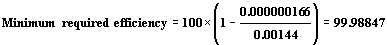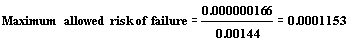To facilitate the choice of the measures, country B will compare the measures through a matrix representing the relationship of the maximum allowed risk of failure and the risk of failure of each measure (Table 4). The threshold value is 1.Any measure with a value smaller then 1 does not provide for the appropriate level of protection and vice-versa.Other methods to compare measures could be used.

Table 4. Parameters of the available measures

 No measure Inspection Treatment Place free Systems approach Area free No trade Efficacy1 0% 90% 99.9998% 99.2% 99.8% 99.9999% 100% Risk (probability) of failure of the measure 1 0.1 0.000002 0.008 0.002 0.000001 0 Measure index2 0 0.001153 57.80 0.0144 0.0576 115.34 ¥

1 Efficacy data is derived from experimentation and sampling (confidence limits should also be considered)

2 Measure index =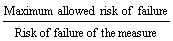Figure 2. Graphical comparison of the measures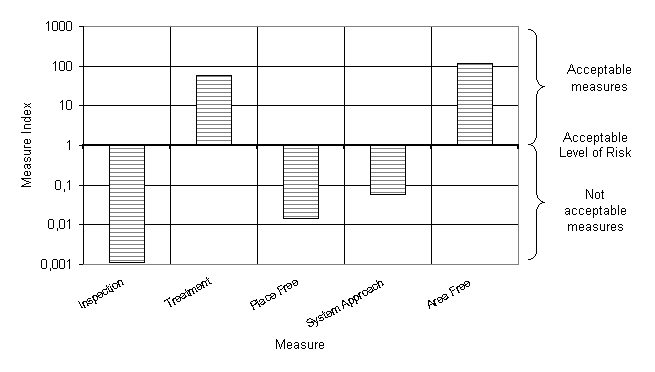5.5. Conclusions

CountryB may accept the import of 20,000 boxes from country A, but should require treatment to achieve the appropriate level of protection.Area freedom would also be acceptable since this would provide even a greater protection level, but it may be viewed as more trade restrictive.

5.6 Alternatives

Suppose that the exporting country has no free areas and that there is no treatment for the pest.The only thing that the exporting country could offer as a phytosanitary measure in this case is a systems approach with the efficiency shown in Table 4.

Following all the steps backward, country B estimates that the levelof protection it will achieve would correspond to one introduction every 15 years.However, the appropriate level of protection could be attained with the system approach if country B authorizes importation of only 1,000 boxes each year.Also if country A increases the efficacy of its systems approach up to the minimum required efficicacy (99.98847) the appropriate level of protection established by country B will be achieved.

6. Summary of the concepts and definitions introduced

Following are the main concepts introduced in this paper and definitions proposed for each.

 Efficacy (of a phytosanitary measure) Percentage of the pest population that is removed from a product by a phytosanitary measure Level of protection Log10 of the product introduction period Maximum allowable risk of failure (MAR) The maximum level of failure for a phytosanitary measure which achieves the acceptable level of risk Minimum required efficacy (MRE) Minimum efficacy of a phytosanitary measure required to achieve the acceptable level of risk Product introduction period Expected period of time between the mean probability of two introductions resulting from the importation of all units of one product from one origin. Mathematically, the reciprocal of the product risk Product risk Probability of introduction of a pest through the importation of all units of one product, from one origin, during a specified period of time Risk of failure (of a phytosanitary measure) Proportion of a pest population that is not removed from a product by a phytosanitary measure. Unit introduction period Expected period of time between two introductions resulting from the importation of one unit of the product. Mathematically, the reciprocal of the unit risk Unit risk Probability of introduction of a pest through the importation of one unit of the product during a specific period of time

7.Graphical Summary of the Relationships

Figure 3 is a graphical demonstration of the relationship between the maximum allowable risk of failure of the phytosanitary measure, the probability of introduction (as estimated by PRA), and the appropriate level of protection

Figure 3.Relationship between the risk of failure, probability of introduction and appropriate level of protection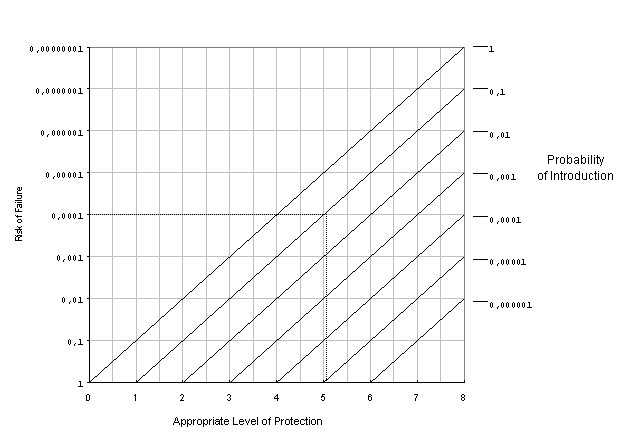The dotted line in the graph is to show that for an appropriate level of protection equal to 5 and a probability of introduction equal to 0.1, it would be necessary to have a phytosanitary measure with a risk of failure less than 0.0001 (or efficacy greater then 99.99 %).

Note: as this graph was constructed based on one unit of the product, the risk of failure must be divided by the total number of units to be imported.

Many thanks to Robert Griffin (USDA) for the final revision.

It should be noted that a negative level of protection means that more then one introduction per time unit is expected.